This is a crosspost from   Cleve’s Corner: Cleve Moler on Mathematics and Computing Cleve Moler is the author of the first MATLAB, one of the founders of MathWorks, and is currently Chief Mathematician at the company. He writes here about MATLAB, scientific computing and interesting mathematics.. See the original post here.

# Trio, A Wooden Puzzle from the Czech Republic

"Clever Toys" is a puzzle company in the Czech Republic. Their Web site describes five different hand-made, wooden puzzles that are related mathematically to the Rubik's Cube.

### Contents

#### Puzzle

Here is the photo of the puzzle "Trio".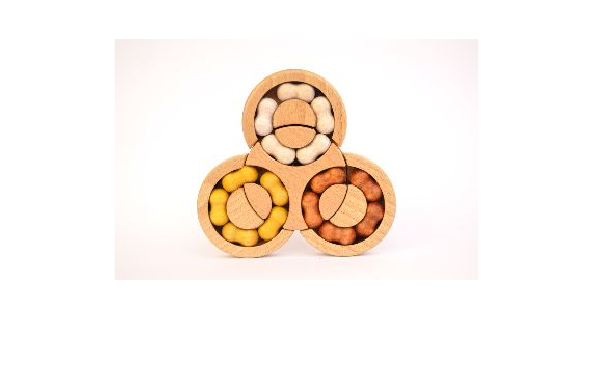Here is an English translation of their description.

The task of this 2D puzzle is to assemble all the ovals according to their color into a basic assembly. By moving the balls in the grooves and turning the center wheel, you can gradually get each ball where you need it.

I am not sure that Clever Toys is still in business. They have not responded to my emails and I have not been able to purchase an actual Trio puzzle. Of course, that is all the motivation I need to make a MATLAB model.

#### Model

Here is the initial configuration. There are three fixed outer, partial, discs and one inner, full, disc. Each of the outer discs has a channel containing 10 marbles. When the inner disc is positioned properly, the marbles in a channel can be rotated. Rotating the inner disc itself moves some, but not all, of the marbles, thereby scrambling the colors.

Like Rubik's Cube, the objective of the puzzle is to return to this initial "solved" state.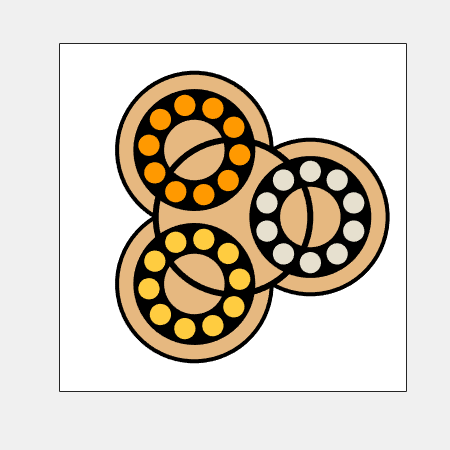Mathematically, both Rubik's Cube and Trio are ultimately based upon rotation matrices. Rubik's Cube is a 3-D puzzle whose state is specified by the position and orientation of 27 cubelets; this gives Rubik's Cube about 4.3*10^19 possible positions. At each step, there are six faces and 12 possible rotations.

Trio is a 2-D puzzle whose state is specified by the colors of the marbles. There are 10 marbles of each of three colors; this implies that Trio has 30!/(10!)^3 = 5.6*10^12 possible positions. At each step, there are eight possible rotations, four discs, clockwise or counter-clockwise.

#### Scramble

A "scramble" is an integer vector with elements between -4 and +4 that specify moves or rotations. Move 0 initializes the model. Move d with d equal to 1, 2, or 3 rotates all the marbles in the d-th channel counter-clockwise for one-tenth of a full rotation. Move d with d equal to -1, -2, or -3 is the corresponding clockwise rotation. Moves -4 and 4 turn the central disc clockwise or counter-clockwise for one-third of a full rotation. This rotates some, but not all, of the marbles and mixes the colors.

Here is a scramble of length 29 that provides our example.

D = [ 4 1 3 1 1 -2 3 1 4 4 2 4 4 -3 -3 -2 -3 -3 2 1 4 -1 3 -4 2]

And here is the scrambled result.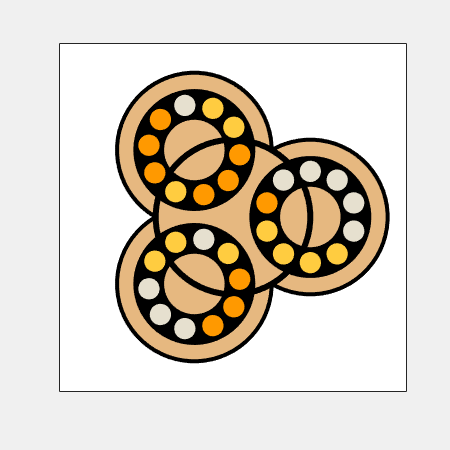#### Animation

This animated gif shows the scrambling process one move at a time. The animation does not repeat automatically, so to start it over again, refresh your browser. If it still doesn't move, find another browser.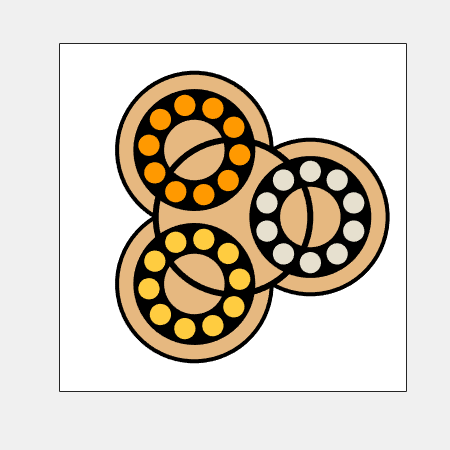#### Unscramble

Reverse the scramble by running it backwards, changing the sign of each move. This will return the scrambled position to the initial position. I call this "unscramble"; it solves the scrambled position by a "follow the breadcrumbs" algorithm.

Both animations take a long time to run -- about 80 seconds with my browser.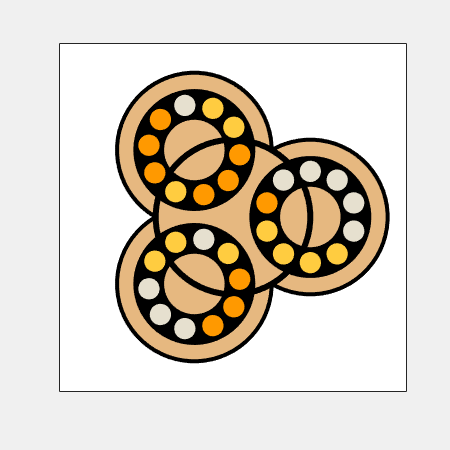#### Solutions

I don't have any idea about how to actually solve a given position without using knowledge of how it was generated, and I don't have any idea about how to quantify the difficulty of finding a solution. This is in sharp contrast to Rubik's Cube where there are measures of difficulty and algorithms for finding optimum solutions.

#### Software

The program available at this link is interactive. Click or alt-click in any one of the four discs to make a move in that disc.

#### Thanks

Thanks to Steve Eddins and Tom Lane for help with this post.

Get the MATLAB code

Published with MATLAB® R2022b